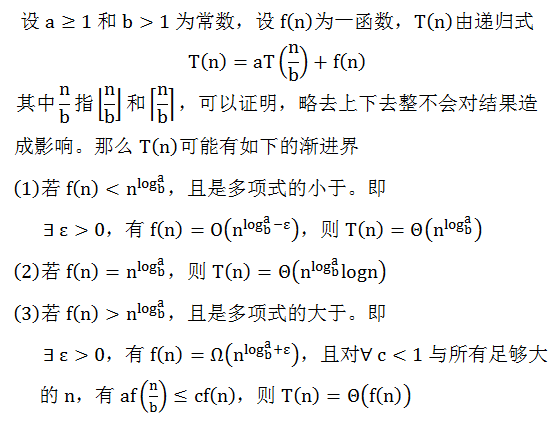# 根据主定理求递归时间复杂度

1. 题目

已知某程序的时间复杂度的递推公式为：T(n)=25T(n/5)+n^2，求T(n)？

2：主定理3. 分析

根据主定理，题目中的f(n)=n^2正好第二种情况，结果为T(n)=(n^2) * logn
如果题目稍作修改：T(n)=25T(n/5)+n^3，则为第三种情况，结果为n^3
T(n)=25T(n/5)+n^1.5，则为第一种情况，结果为n^2

4. 参考

主定理和递归式复杂度分析    http://blog.kingsamchen.com/archives/524